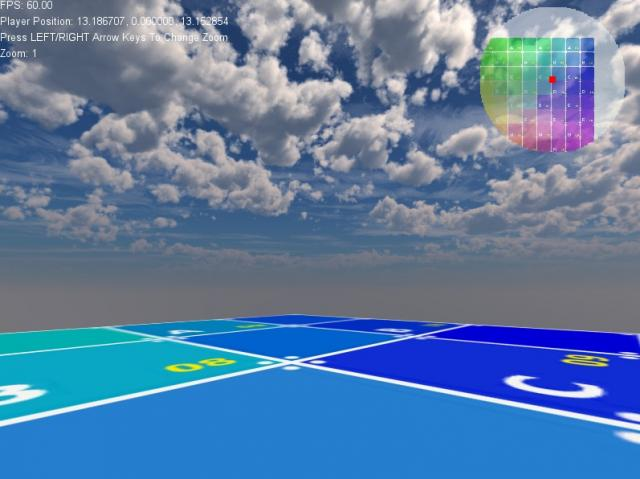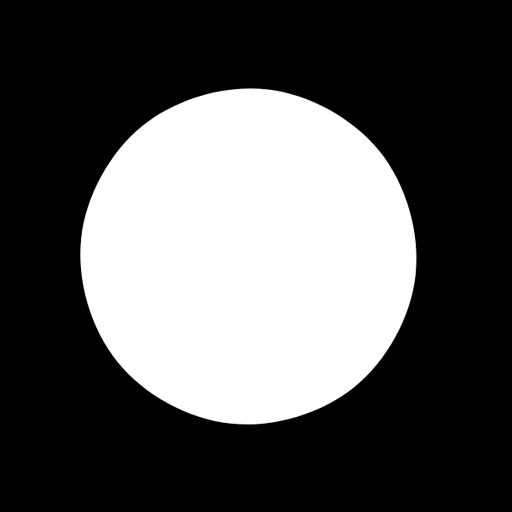Recommended Posts

I am thinking of creating a minimap generator.

I was wondering if anyone had suggestions on how to place the camera?

You have a map of X by X size (512, 1024, 2048)

You need to generate an image of Y b Y size (probably 2048)

Position of the camera would be at Vec3(0.0, Q, 0.0)

Where Q is the height of the camera.

To have the projection where the map bounds end at the same bounds of the context, what height would you have to set your camera at?

Is this just simple trigonometry?

Q = X/Math::tan(camera->GetFOV()); ?

I had played around with this at one time and don't quite remember everything i tried and why I ended up with the code i did, but this was the example code I had left it at:

mm_pos = math.tan((90-camera:GetFOV()/2)*math.pi/180) * (mm_size/2)

example script:

window = Window:Create("example",0,0,800,600,Window.Titlebar+Window.Center)
context = Context:Create(window)
world = World:Create()
world:SetSkybox(skybox)
light = DirectionalLight:Create()
light:SetRotation(45,45,0)
window:HideMouse()

player:SetPosition(0,6,0)
camera = tolua.cast(player.script.camera, "Camera")
camera:SetMultisampleMode(8)

mm_size = 64.0 -- size of map
mm_zoom = 0.1
mm_toggle = 0
mm_shader:SetVec2("terrain_size", Vec2(mm_size,mm_size)) --send terrain size to shader uniform
cur_buffer = Buffer:GetCurrent()
mm_buffer = Buffer:Create(1024,1024,1,0) -- can be any size but higher = better resolution

terrain = Model:Box()
terrain:SetScale(mm_size,1,mm_size)
terrain:SetPosition(0,-0.5,0)
terrain:SetMaterial(terrainmat)
shape = Shape:Box(0,0,0, 0,0,0, mm_size,1,mm_size)
terrain:SetShape(shape)
shape:Release()

while not window:KeyHit(Key.Escape) do
if window:Closed() then return false end

Time:Update()
world:Update()
world:Render()

if mm_toggle==0 then
mm_toggle = 1
camera_prev_mat = camera:GetMatrix()
--calculate proper height based on camera's FOV
mm_pos = math.tan((90-camera:GetFOV()/2)*math.pi/180) * (mm_size/2)
camera:SetPosition(0,mm_pos,0)
camera:SetRotation(90,0,0)
mm_buffer:Enable()
world:Render()
minimap = mm_buffer:GetColorTexture(0)
camera:SetMatrix(camera_prev_mat)
cur_buffer:Enable()
end

if window:KeyHit(Key.Right) then mm_zoom = mm_zoom + 0.1 end
if window:KeyHit(Key.Left) then mm_zoom = mm_zoom - 0.1 end
mm_zoom = math.max(mm_zoom, 0.1)
mm_zoom = math.min(mm_zoom, 1.0)

context:SetBlendMode(1)
context:SetColor(1,1,1,0.55)
pos = player:GetPosition(true)
mm_shader:SetVec2("player_pos", Vec2(pos.x,pos.z)) --send player's position to shader uniform
mm_shader:SetVec2("zoom", Vec2(mm_zoom)) --send current zoom to shader uniform
minimap:Bind(1)
context:SetColor(1,0,0,1)
context:DrawRect(686,96,8,8,0,0)
context:SetColor(1,1,1,1)
context:DrawText(string.format("FPS: %.2f", Time:UPS()),2,2)
context:DrawText("Player Position: "..player:GetPosition():ToString(),2,22)
context:DrawText("Press LEFT/RIGHT Arrow Keys To Change Zoom",2,42)
context:DrawText("Zoom: "..mm_zoom,2,62)
context:SetBlendMode(0)
context:Sync(true)
end

•2

For a minimap I would actually use an orthographic projection mode. I guess, in an orthographic projection, you can simply set the width and the height of the camera to exactly your map's dimensions.

If you want to use a perspective projection:

macklebee's formula surely looks nice but the map doesn't include the whole map (which I suppose, you want to have).

Is this just simple trigonometry?

Q = X/Math::tan(camera->GetFOV()); ?

Doing the math I arrive at

Q = X/(2*Math::tan(camera->GetFOV()/2.0f))

(though I didn't test it)

If you want to use a perspective projection:

macklebee's formula surely looks nice but the map doesn't include the whole map (which I suppose, you want to have).

Actually it does give the whole map. You do not see the whole map in the minimap because I implemented in the minimap's shader the ability to zoom in/out and a masked circle. Also, if you look at the code i posted you will see that the minimap is only rendered once - so yes, it does render the entire map or I would not be able to see the edges at all.Oh, my apologies then.

I've been trying to make sense of the math you used, but I actually don't get how that's supposed to work or what my formula does wrong then. I get that "math.pi/180" term is only for deg->rad, as the standard math.tan-function works with rad, whereas the leadwerks-Math::rad-function works with deg, but for the rest, you have "tan(90-fov/2)", where I have "1/tan(fov/2)", and these terms are surely not identical...

No matter how I draw things out, I always end up with the term I wrote above.Thanks for actually drawing that thing out. So you're calculating with theta, while I'm calculating directly with the angle fov/2.0. So that's where that difference comes from. I actually checked back with wolfram-alpha and actually both of our terms are equal. When I first checked it, I forgot that wolfram-alpha uses rad and I had to transform the 90 before checking...So... now at least I can sleep in peaceOn 9/15/2019 at 6:40 AM, Marcousik said:

Just a question: How do you get the minimap to be round ???

and what is exactly mm_mask.tex (did not find it)

He mentions a "masked circle" in his second post.  I suspect that's the answer, that mask.tex is the circle mask for the mini map.

If you look at the shader code, you see this:

if (mask.r > 0.0) {
fragData0 = drawcolor * texture(texture1, (vTexCoords0 * zoom) + UV - zoom * 0.5);
}
else {
}

which looks like it discards (doesn't draw) pixels that have a red value of 0, if I understand it right.

On 2/5/2017 at 12:18 AM, macklebee said:

I implemented in the minimap's shader the ability to zoom in/out and a masked circle

He is speaking about implemented thing... So I don't know, and no idea how  mask.tex looks like...

this is very complicated for me, I find this a total genius code, it would be worse making a tutorial on this, on how you have to use buffer...

maybe can macklabee throw a bit light on this ?

I do not understand why should a red va﻿lue of o be discarded.

Anyway thx for the help

A lot of it is above my head too (since I don't know shaders) but it seems like he uses black as a transparent value (that's why the value of red is 0), anything else as drawable.  So your mask could look something like this:Join the conversation

You can post now and register later. If you have an account, sign in now to post with your account.Reply to this topic...

×   Pasted as rich text.   Paste as plain text instead

Only 75 emoji are allowed.

×   Your previous content has been restored.   Clear editor

×   You cannot paste images directly. Upload or insert images from URL.

×
• Recent Topics

• Lets make a game?

By UberReady, in General Discussion

• 5 replies
• 290 views
• My latest work (scifi textures 2.0) - scifi materials 1 2 3 4 6

By TWahl, in Game Art

• 133 replies
• 10,124 views
• One more try? ;(

By 💎Yue💎, in Showcase

• 3 replies
• 46 views
• How do you access imported terrain in code?

By havenphillip, in Programming

• 3 replies
• 108 views
• Question: Code Blocks on Windows??

By 💎Yue💎, in Technical Assistance

• Has best answer